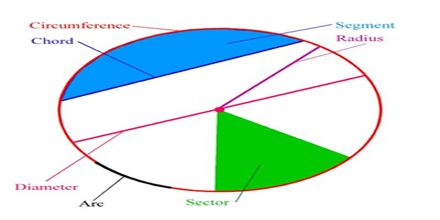Mathematic

# Circle TerminologyPrime purpose of this lecture is to present on Circle Terminology. Some common Circle Terminology are describe in this lecture. Radius (or Radii for plural): The segment joining the center of a circle to a point on the circle. Diameter: A chord that passes through the center of a circle. Chord: A segment joining two points on a circle. Secant: A line that intersects the circle at exactly two points. Tangent: A line that intersects a circle at exactly one point. Central Angle: An angle whose vertex is at the center of a circle. Inscribed Angle: An angle whose vertex is on a circle and whose sides are determined by two chords.